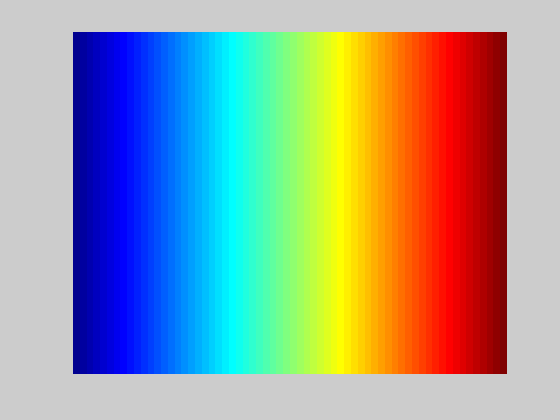I was not aware of the fact that the color spectrum used in many charts are called the “Jet palette”, named after how MATLAB calls it. This palette also available in matplotlib so it is quite ubiquitous. According to a post, the spectrum is based on a linear blending between the following RGB colors:

#00007F: dark blue
#0000FF: blue
#007FFF: azure
#00FFFF: cyan
#7FFF7F: light green
#FFFF00: yellow
#FF7F00: orange
#FF0000: red
#7F0000: dark redFrom the GIMP version of the palette we know that these 9 colors are evenly positioned across the spectrum. According to stackoverflow, the following function in C (attributed to Paul Bourke) computes the color:

/*
Return a RGB colour value given a scalar v in the range [vmin,vmax]
In this case each colour component ranges from 0 (no contribution) to
1 (fully saturated), modifications for other ranges is trivial.
The colour is clipped at the end of the scales if v is outside
the range [vmin,vmax]
*/

typedef struct {
double r,g,b;
} COLOUR;

COLOUR GetColour(double v,double vmin,double vmax)
{
COLOUR c = {1.0,1.0,1.0}; // white
double dv;

if (v < vmin)
v = vmin;
if (v > vmax)
v = vmax;
dv = vmax - vmin;

if (v < (vmin + 0.25 * dv)) {
c.r = 0;
c.g = 4 * (v - vmin) / dv;
} else if (v < (vmin + 0.5 * dv)) {
c.r = 0;
c.b = 1 + 4 * (vmin + 0.25 * dv - v) / dv;
} else if (v < (vmin + 0.75 * dv)) {
c.r = 4 * (v - vmin - 0.5 * dv) / dv;
c.b = 0;
} else {
c.g = 1 + 4 * (vmin + 0.75 * dv - v) / dv;
c.b = 0;
}

return(c);
}


According to the GIMP palette specification, the color change is linear in the RGB space. For the 9 colors, the whole spectrum are divided into 8 equal sections and we can interpolate according to the colors above. However, there is a simpler method, by realizing the color in each channel has only one peak from the beginning to the end. Take the green channel as an example, its peak is at the center of the spectrum, which plateau at max intensity for 1/4 of the spectrum and outside of the plateau fall to min intensity for 1/4 of the spectrum on both sides. The total signal width is 3/4 of the spectrum.

With the blue, green, and red peaked at 25%, 50%, and 75% position respectively, the following python code is how we can compute the RGB value for the range of [0,1]:

def clip(v, minv, maxv):
return max(minv, min(maxv, v))

def triangle(t, peak):
"""Compute the triangle impulse, of range 0 to 1.5, at t, with signal width
of 3/4, then clip the output to [0,1]

Returns:
signal intensity in range of [0,1]
"""
halfwidth = 3/8
maxval = 1.5
return clip(maxval*(1 - abs(peak-t)/halfwidth), 0, 1)

def jet_rgb(v):
"""Compute the jet colormap
Args:
v: float of range [0,1]
Returns:
Tuple of 3 floats, each in the range of [0,1], for the RGB intensities
"""
R = triangle(v, 0.75)
G = triangle(v, 0.50)
B = triangle(v, 0.25)
return (R,G,B)